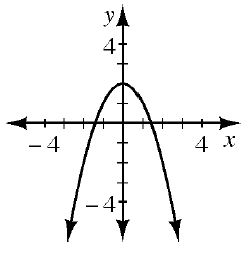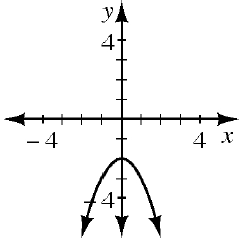### Home > CCA > Chapter 8 > Lesson 8.1.4 > Problem8-44

8-44.

Match each rule below with its corresponding graph. Can you do this without making any tables? Explain your selections.

1. $y=-x^2-2$

1. $y=x^2-2$

1. $y=-x^2+2$

1.1.1.Determine the direction of the opening and $y$-intercept for each of the given equations.

$2$